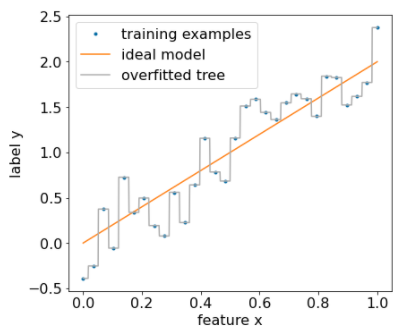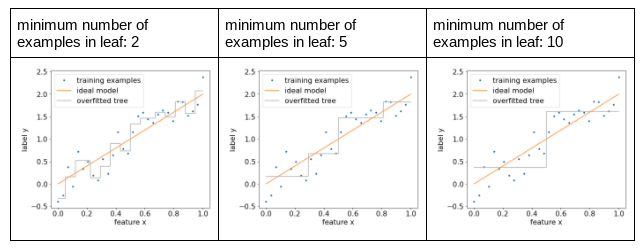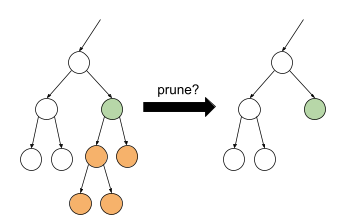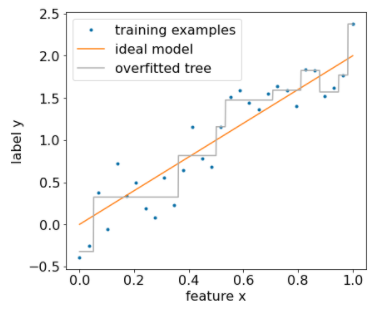## Overfitting and pruning

Using the algorithm described above, we can train a decision tree that will perfectly classify training examples, assuming the examples are separable. However, if the dataset contains noise, this tree will overfit to the data and show poor test accuracy.

The following figure shows a noisy dataset with a linear relation between a feature x and the label y. The figure also shows a decision tree trained on this dataset without any type of regularization. This model correctly predicts all the training examples (the model's prediction match the training examples). However, on a new dataset containing the same linear pattern and a different noise instance, the model would perform poorly.Figure 12. A noisy dataset.

To limit overfitting a decision tree, apply one or both of the following regularization criteria while training the decision tree:

• Set a maximum depth: Prevent decision trees from growing past a maximum depth, such as 10.
• Set a minimum number of examples in leaf: A leaf with less than a certain number of examples will not be considered for splitting.

The following figure illustrates the effect of differing minimum number of examples per leaf. The model captures less of the noise.Figure 13. Differing minimum number of examples per leaf.

You can also regularize after training by selectively removing (pruning) certain branches, that is, by converting certain non-leaf nodes to leaves. A common solution to select the branches to remove is to use a validation dataset. That is, if removing a branch improves the quality of the model on the validation dataset, then the branch is removed.

The following drawing illustrates this idea. Here, we test if the validation accuracy of the decision tree would be improved if the non-leaf green node was turned into a leaf; that is, pruning the orange nodes.Figure 14. Pruning a condition and its children into a leaf.

The following figure illustrates the effect of using 20% of the dataset as validation to prune the decision tree:Figure 15. Using 20% of the dataset to prune the decision tree.

Note that using a validation dataset reduces the number of examples available for the initial training of the decision tree.

Many model creators apply multiple criteria. For example, you could do all of the following:

• Apply a minimum number of examples per leaf.
• Apply a maximum depth to limit the growth of the decision tree.
• Prune the decision tree.
In TF-DF, the learning algorithms are pre-configured with default values for all the pruning hyperparameters. For example, here are the default values for two pruning hyperparameters:
• The minimum number of examples is 5 (min_examples = 5)
• 10% of the training dataset is retained for validation (validation_ratio = 0.1).
You can disable pruning with the validation dataset by setting validation_ratio=0.0.

Those criteria introduce new hyperparameters that need to be tuned (e.g. maximum tree depth), often with automated hyperparameter tuning. Decision trees are generally fast enough to train to use hyperparameter tuning with cross-validation. For example, on a dataset with "n" examples:

• Divide the training examples into p non-overlapping groups. For example: p=10.
• For all the possible hyperparameter values; for example, max depth in {3,5,6,7,8,9}, min examples in {5,8,10,20}.
• Evaluate, on each group, the quality of a decision tree trained on the other p-1 groups.
• Select the hyperparameter value with the best averaged evaluation.
• Train a final decision tree using all the "n" examples with the selected hyperparameters.

In this section we discussed the ways decision trees limit overfitting. Despite these methods, underfitting and overfitting are major weaknesses of decision trees. Decision forests introduce new methods to limit overfitting, which we will see later.

## Direct decision tree interpretation

Decision trees are easily interpretable. That said, changing even a few examples can completely change the structure—and therefore the interpretation—of the decision tree.

Because of the way decision trees are built, partitioning the training examples, one can use a decision tree to interpret the dataset itself (as opposed to the model). Each leaf represents a particular corner of the dataset.

In TF-DF, you can plot decision trees with the tfdf.model_plotter.plot_model_in_colab() function.

However, indirect interpretation is also informative.

[{ "type": "thumb-down", "id": "missingTheInformationINeed", "label":"Missing the information I need" },{ "type": "thumb-down", "id": "tooComplicatedTooManySteps", "label":"Too complicated / too many steps" },{ "type": "thumb-down", "id": "outOfDate", "label":"Out of date" },{ "type": "thumb-down", "id": "samplesCodeIssue", "label":"Samples / code issue" },{ "type": "thumb-down", "id": "otherDown", "label":"Other" }]
[{ "type": "thumb-up", "id": "easyToUnderstand", "label":"Easy to understand" },{ "type": "thumb-up", "id": "solvedMyProblem", "label":"Solved my problem" },{ "type": "thumb-up", "id": "otherUp", "label":"Other" }]